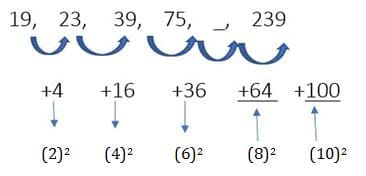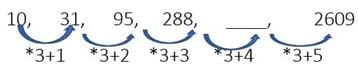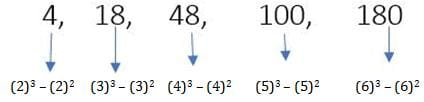CAT  >  Number Series Notes - 1

# Number Series Notes - 1 Notes - CAT

## Document Description: Number Series Notes - 1 for CAT 2022 is part of CAT preparation. The notes and questions for Number Series Notes - 1 have been prepared according to the CAT exam syllabus. Information about Number Series Notes - 1 covers topics like Introduction and Number Series Notes - 1 Example, for CAT 2022 Exam. Find important definitions, questions, notes, meanings, examples, exercises and tests below for Number Series Notes - 1.

Introduction of Number Series Notes - 1 in English is available as part of our CAT preparation & Number Series Notes - 1 in Hindi for CAT courses. Download more important topics, notes, lectures and mock test series for CAT Exam by signing up for free. CAT: Number Series Notes - 1 Notes - CAT
 1 Crore+ students have signed up on EduRev. Have you?

Sequences and series (as the name suggests) consists of questions where you are supposed to understand the logic behind a given sequence or series of number/alphabets. Based on this understanding you are supposed to determine either:

• A continuation to the series
• The immediate next term of the series or
• A missing term/terms within the series.

While preparing yourself to solve such questions, you should improve your ability to spot a particular relationship between terms within the series. The common logical premises used for questions are given here.

Introduction

Number series is a form of sequence, where some numbers are mistakenly put into the series of numbers and some number is missing in that series, we need to observe first and then find the accurate number to that series.

Sequence is the list of numbers written in the specific order.

For ex.  1, 4, 9, 16, 25, 36……..

Some Common types and Their TricksType 1 - Addition / Subtraction or Multiplication / Division
Example 1:  19, 23, 39, 75, _, 239

Most common trick to solve a number series is to solve by checking difference between two adjacent numbers, but difference could not only lead to addition/subtraction but it can also be with multiplication/division.
Therefore, to check whether to think addition wise or multiplication wise in increasing sequence one must make assumption with the help of difference between first and last number of the given sequence.

• If the difference seems to be less according to the number of steps used to make last number from first than we should check addition
• If the difference seems to be large or too large one must check multiplication trick between the adjacent numbers

In the Exercise 1. given above, difference between first no. (19) and last number (239) is 220.
Now a question will arise how we will assume whether the difference is more or less. We will assume it by keeping in mind number of steps required to start from first number till last number.
In Example 1, 19 becomes 239 in five steps, because there are four more numbers between them, one of which we have to find out.
Difference of 220 between 19 and 239 in five steps logically giving priority to addition over multiplication in an increasing sequence like this.75 + 64 = 139, 139 + 100 = 239 139 is correct answer.

Question for Number Series Notes - 1
Try yourself:Look at this series: 7, 10, 8, 11, 9, 12, ... What number should come next?

Example 2: 10, 31, 95, 288, ___, 2609
As we can see in above ex. Difference between first number (10) and last number (2609) is 2599 in five steps, which indicate us to check multiplication trick between the numbers.(288*3) + 4 = 868, is the correct answer.
Note: While checking multiplication trick always start from right end of the sequence.

Example 3: 30, 34, 43, 59, 84, 120,?
(a) 169
(b) 148
(c) 153
(d) 176
(e) None of these
Ans. (a)
Solution: The given pattern is: +22, 32, +42, + 62, +72
So, missing term is 169 = 120 + 72

Example 4: 40, 54, 82, ?, 180 ,250
(a) 142
(b) 124
(c) 136
(d) 163
(e) None of these

Ans. (b)
Solution: The pattern is: +14, + 28, + 42, + 52, + 70
So, missing term is 82 + 42 = 124

Question for Number Series Notes - 1
Try yourself:Look carefully for the pattern, and then choose which pair of numbers comes next - 8,11, 21, 15, 18, 21, 22

Example 5: 0, 1, 3, 8, 18, 35, 264
(a) 62
(b) 35
(c) 18
(d) 8
(e) None of these

Ans. (a)
Solution: The pattern is +(02 + 1), +(12 + 1), + (22 + 1) ,+ (32 + 1), + (42 + 1), + (52 + 1)
So, 264 is wrong and must be replaced by 35 + (52+1) = 62

Example 6: 1, 9, 125, 49, 729, 121, 2147
(a) 2147
(b) 729
(c) 125
(d) 1
(e) None of these
Ans.
(a)

Example 7: 5531, 5506, 5425, 5304, 5135, 4910, 4621
(a) 5531
(b) 5425
(c) 4621
(d) 5135
(e) 5506
Ans.
(a)
Solution: The number should be 5555 in place of 5531.
-72, -92, -112, -132, -152, -172…

Example 8: 6, 7, 9, 13, 26, 37, 69
(a) 7
(b) 26
(c) 69
(d) 37
(e) 9
Ans.
(b)
Solution: The number should be 21 in place of 26. +1, +2, +4, +8, +16, +32

Example 9: 1, 3, 10, 36, 152, 760, 4632
(a) 3
(b) 36
(c) 4632
(d) 760
(e) 152
Ans.
(d)
Solution: The number should be 770 in place of 760.
×1 +2, ×2 +4, ×3 +6, ×4 + 8, ×5 +10, ×6 + 12, …

Example 10: 4, 3, 9, 34, 96, 219, 435
(a) 4
(b) 9
(c) 34
(d) 435
(e) 219
Ans.
(d)
Solution: The series is 02+ 4, 12 + 2, 32 + 0, 62 - 2, 102 - 4, 152 - 6, 212 – 8…
Hence, 435 should be replaced with 433

Example 11: 157.5, 45, 15, 6, 3, 2, 1
(a) 1
(b) 2
(c) 6
(d) 157.5
(e) 45

Ans. (a)
Solution: The number should be 2 in place of 1. 3.5, 3, 2.5, 2, 1.5, 1

Type 2 - Perfect Square or Perfect Cube

Question: 4, 18, 48, 100, 180, ___.
In case if Type-1 is not applicable in a sequence, then in the next step we must compare given numbers or their differences to square or cube of natural numbers, as in the above example.Therefore, (7)3-(7)2 = 294, is the correct answer.

1. Perfect Square Series: This Types of Series are based on square of a number which is in same order and one square number is missing in that given series.

Question for Number Series Notes - 1
Try yourself:In the following options, a few number series are present. One of them has an error, pick the wrong one out:

Example 1: 841, ?, 2401, 3481, 4761
Ans. 292, 392, 492, 592, 692

Question for Number Series Notes - 1
Try yourself:In the sequence given below, a term is missing. The missing term is written in the options that are present below. Find the missing term and choose it from the options below: 8, 12, 21, 37, __

2. Perfect Cube Series: This Types of Series are based on cube of a number which is in same order and one cube number is missing in that given series.

Example 1: 3375, ?, 24389, 46656, 79507
Ans. 153, 223, 293, 363, 433

(Each cube digit added with seven to become next cube number)

Example 2: 729, 6859, 24389, ?, 117649, 205379
Ans. 93, 193, 293, 393, 493, 593

Example 3: 1000, 8000, 27000, 64000, ?
Ans. 103, 203, 303, 403, 503

Question for Number Series Notes - 1
Try yourself:In the following series, a number is such that it does not belong to the arrangement.

8000, 27000, 64000, 105000

Select the option that has this number:

The document Number Series Notes - 1 Notes - CAT is a part of CAT category.
All you need of CAT at this link: CAT
 Use Code STAYHOME200 and get INR 200 additional OFF

### How to Prepare for CAT

Read our guide to prepare for CAT which is created by Toppers & the best Teachers

Track your progress, build streaks, highlight & save important lessons and more!

,

,

,

,

,

,

,

,

,

,

,

,

,

,

,

,

,

,

,

,

,

;